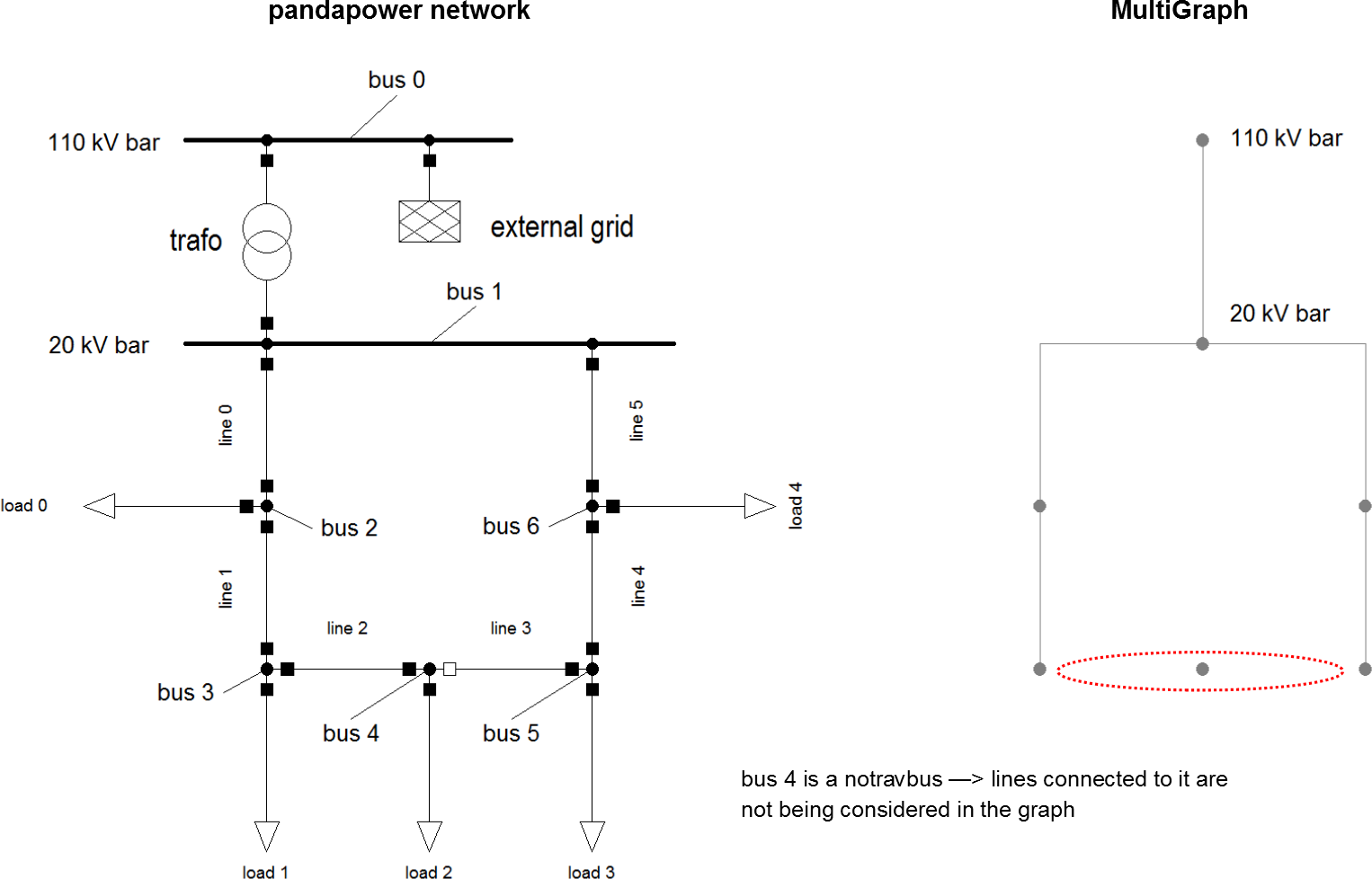# Create networkx graph¶

The basis of all topology functions is the conversion of a padapower network into a NetworkX MultiGraph. A MultiGraph is a simplified representation of a network’s topology, reduced to nodes and edges. Busses are being represented by nodes (Note: only buses with in_service = 1 appear in the graph), edges represent physical connections between buses (typically lines or trafos). Multiple parallel edges between nodes are possible.

This is a very simple example of a pandapower network being converted to a MultiGraph. (Note: The MultiGraph’s shape is completely arbitrary since MultiGraphs have no inherent shape unless geodata is provided.)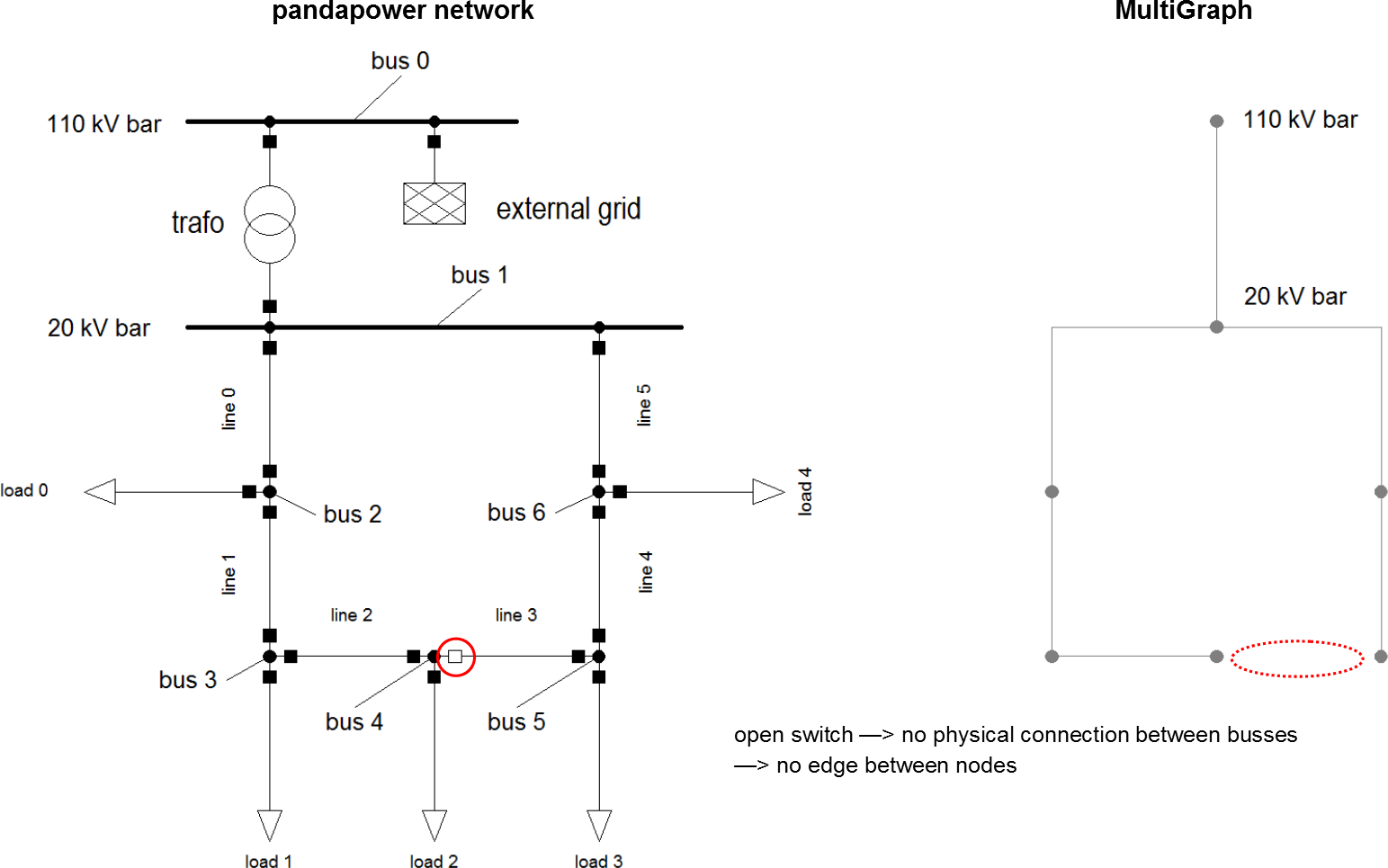Nodes have the same indicees as the buses they originate from. Edges are defined by the nodes they connect. Additionally nodes and edges can hold key/value attribute pairs.

The following attributes get transferred into the MultiGraph:

lines

trafos

• from_bus

• to_bus

• length_km

• index

• in_service

• max_i_ka

• hv_bus

• lv_bus

• index

• in_service

Apart from these there are no element attributes contained in the MultiGraph!

Creating a multigraph from a pandapower network

The function create_nxgraph function from the pandapower.topology package allows you to convert a pandapower network into a MultiGraph:

pandapower.topology.create_nxgraph(net, respect_switches=True, include_lines=True, include_impedances=True, include_dclines=True, include_trafos=True, include_trafo3ws=True, nogobuses=None, notravbuses=None, multi=True, calc_branch_impedances=False, branch_impedance_unit='ohm', library='networkx', include_out_of_service=False)

Converts a pandapower network into a NetworkX graph, which is a is a simplified representation of a network’s topology, reduced to nodes and edges. Busses are being represented by nodes (Note: only buses with in_service = 1 appear in the graph), edges represent physical connections between buses (typically lines or trafos).

INPUT:

net (pandapowerNet) - variable that contains a pandapower network

OPTIONAL:
respect_switches (boolean, True) - True: open switches (line, trafo, bus) are being considered (no edge between nodes)

False: open switches are being ignored

include_lines (boolean or index, True) - determines, whether or which lines get

converted to edges

include_impedances (boolean or , True) - determines, whether or which per unit

impedances (net.impedance) are converted to edges

include_dclines (boolean or index, True) - determines, whether or which dclines get

converted to edges

include_trafos (boolean or index, True) - determines, whether or which trafos get

converted to edges

include_trafo3ws (boolean or index, True) - determines, whether or which trafo3ws get

converted to edges

nogobuses (integer/list, None) - nogobuses are not being considered in the graph

notravbuses (integer/list, None) - lines connected to these buses are not being

considered in the graph

multi (boolean, True) - True: The function generates a NetworkX MultiGraph, which allows

multiple parallel edges between nodes False: NetworkX Graph (no multiple parallel edges)

calc_branch_impedances (boolean, False) - determines wether impedances are calculated

and added as a weight to all branches or not. Impedances can be added in ohm or per unit (see branch_impedance unit parameter). DC Lines are considered as infinity.

branch_impedance_unit (str, “ohm”) - defines the unit of the branch impedance for

calc_branch_impedances=True. If it is set to “ohm”, the parameters ‘r_ohm’, ‘x_ohm’ and ‘z_ohm’ are added to each branch. If it is set to “pu”, the parameters are ‘r_pu’, ‘x_pu’ and ‘z_pu’.

include_out_of_service (bool, False) - defines if out of service buses are included in the nx graph

OUTPUT:

mg - Returns the required NetworkX graph

EXAMPLE:

import pandapower.topology as top

mg = top.create_nx_graph(net, respect_switches = False) # converts the pandapower network “net” to a MultiGraph. Open switches will be ignored.

Examples

create_nxgraph(net, respect_switches = False)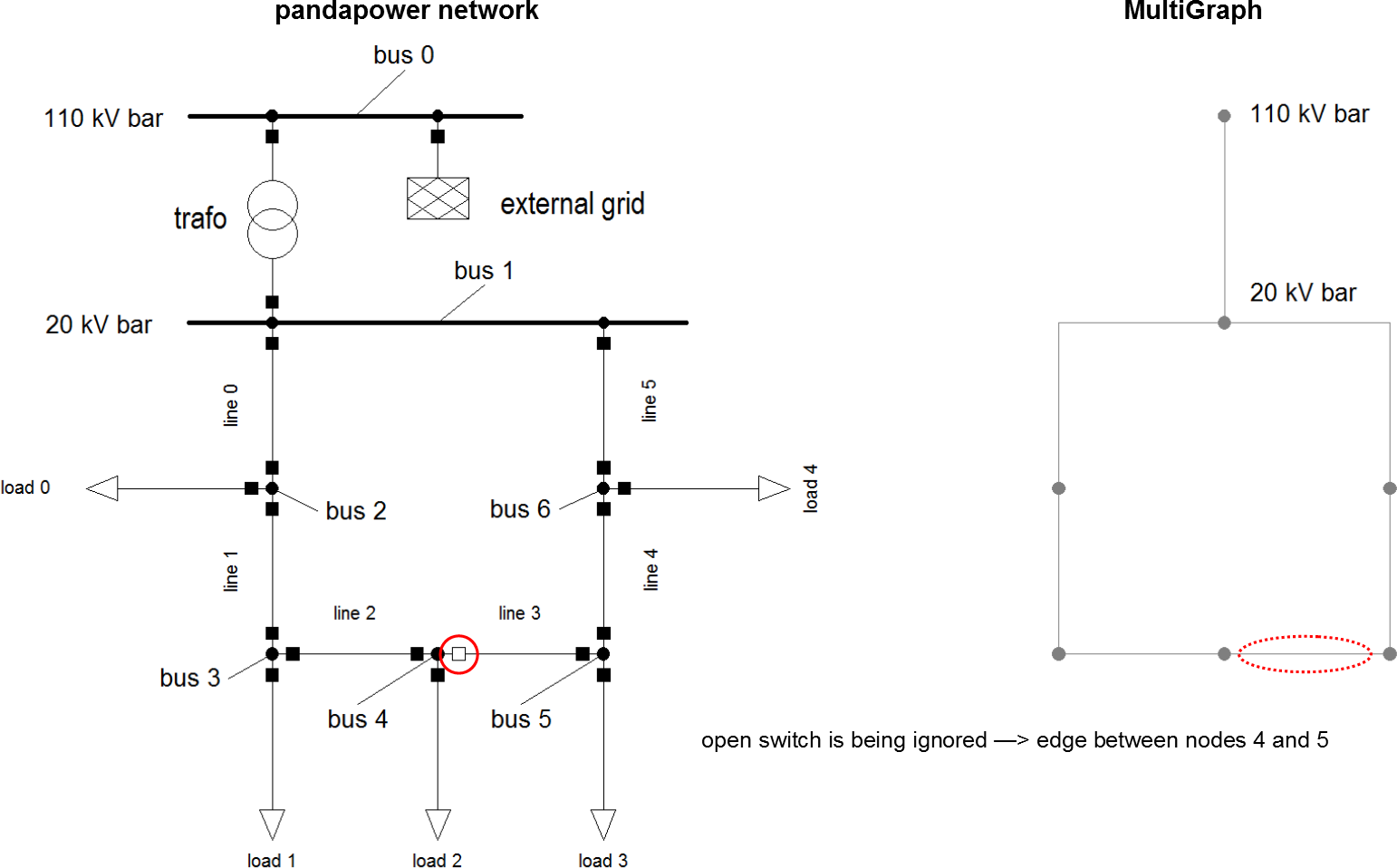create_nxgraph(net, include_lines = False, include_impedances = False)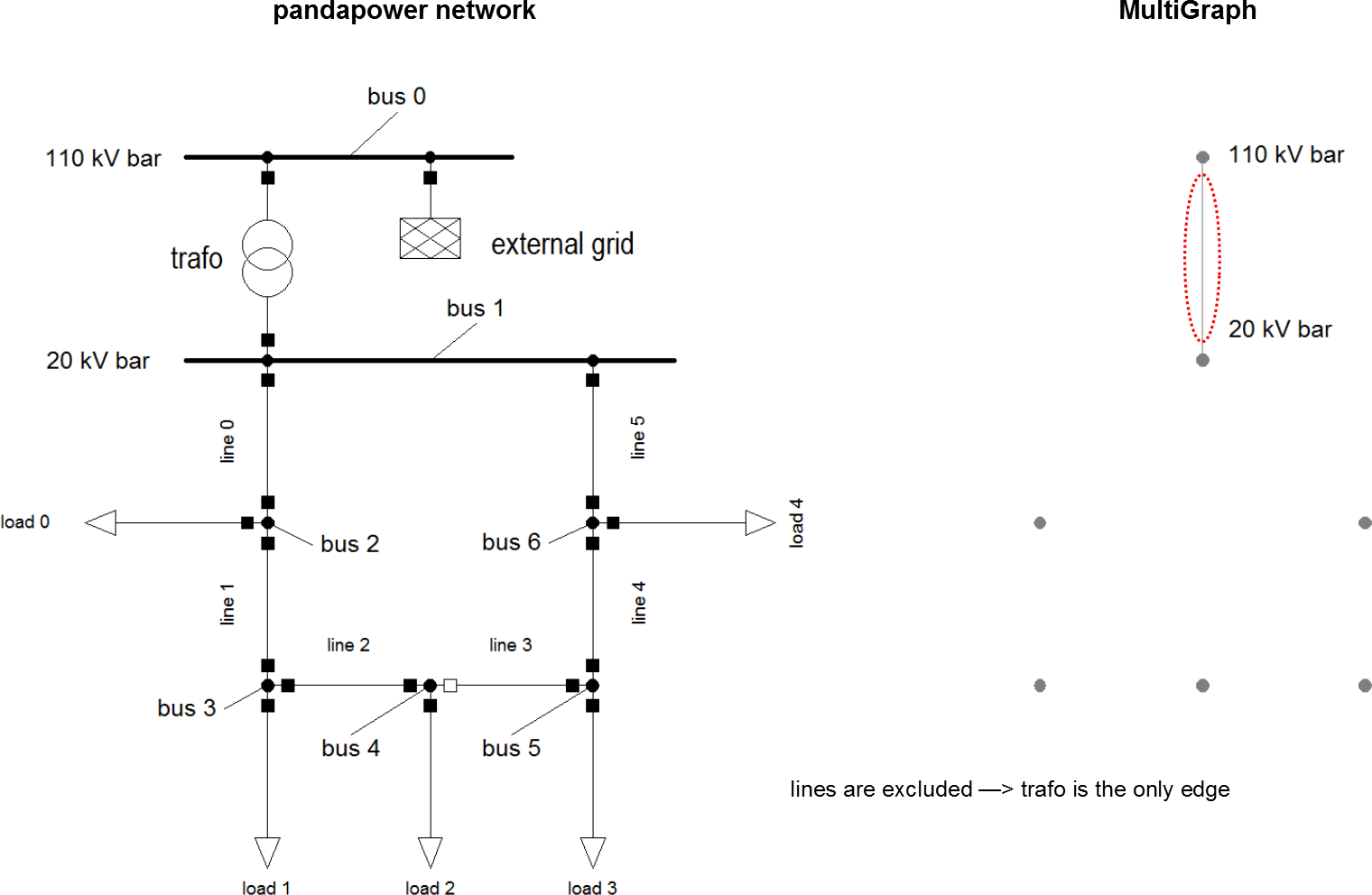create_nxgraph(net, include_trafos = False)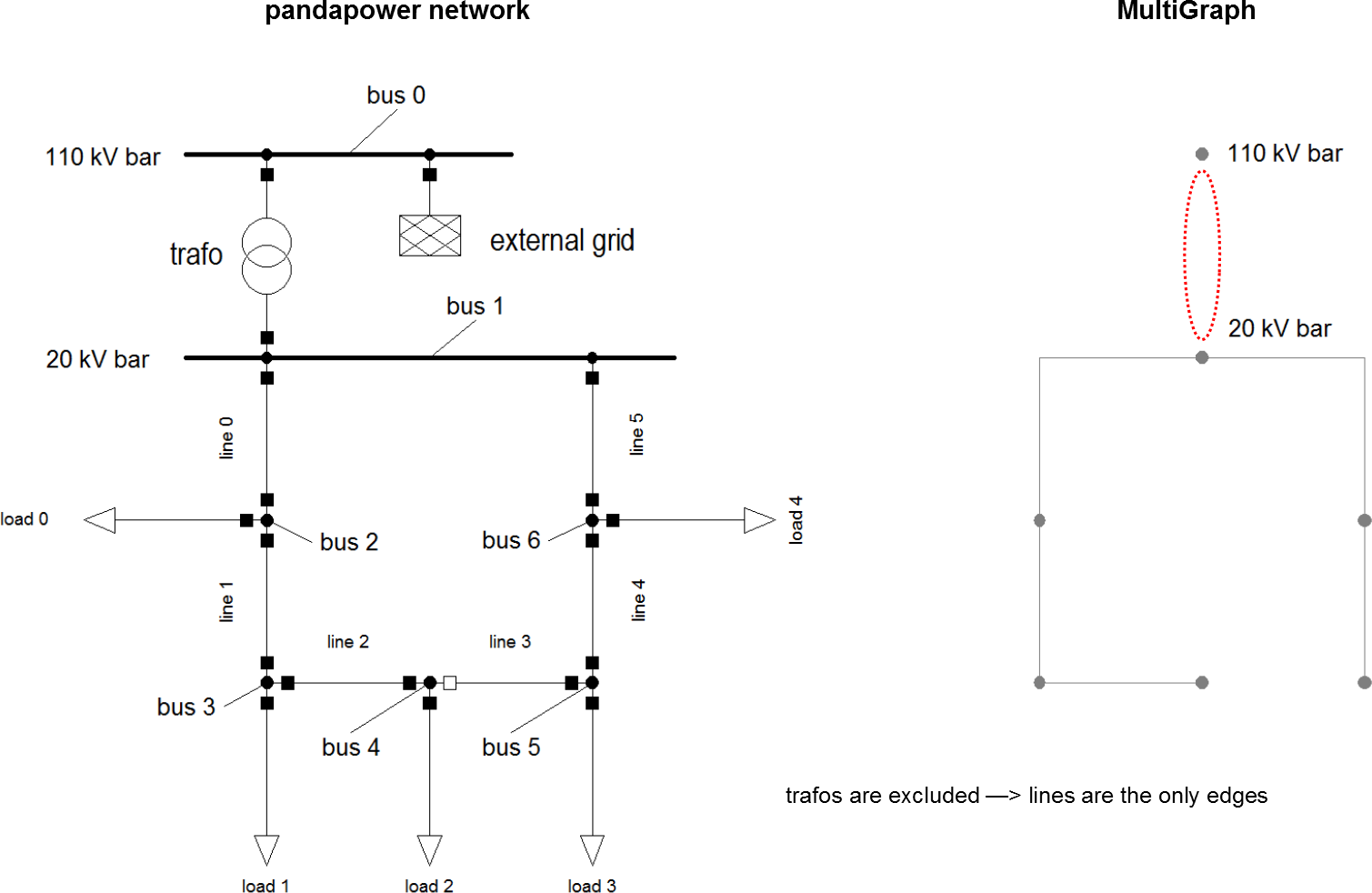create_nxgraph(net, nogobuses = )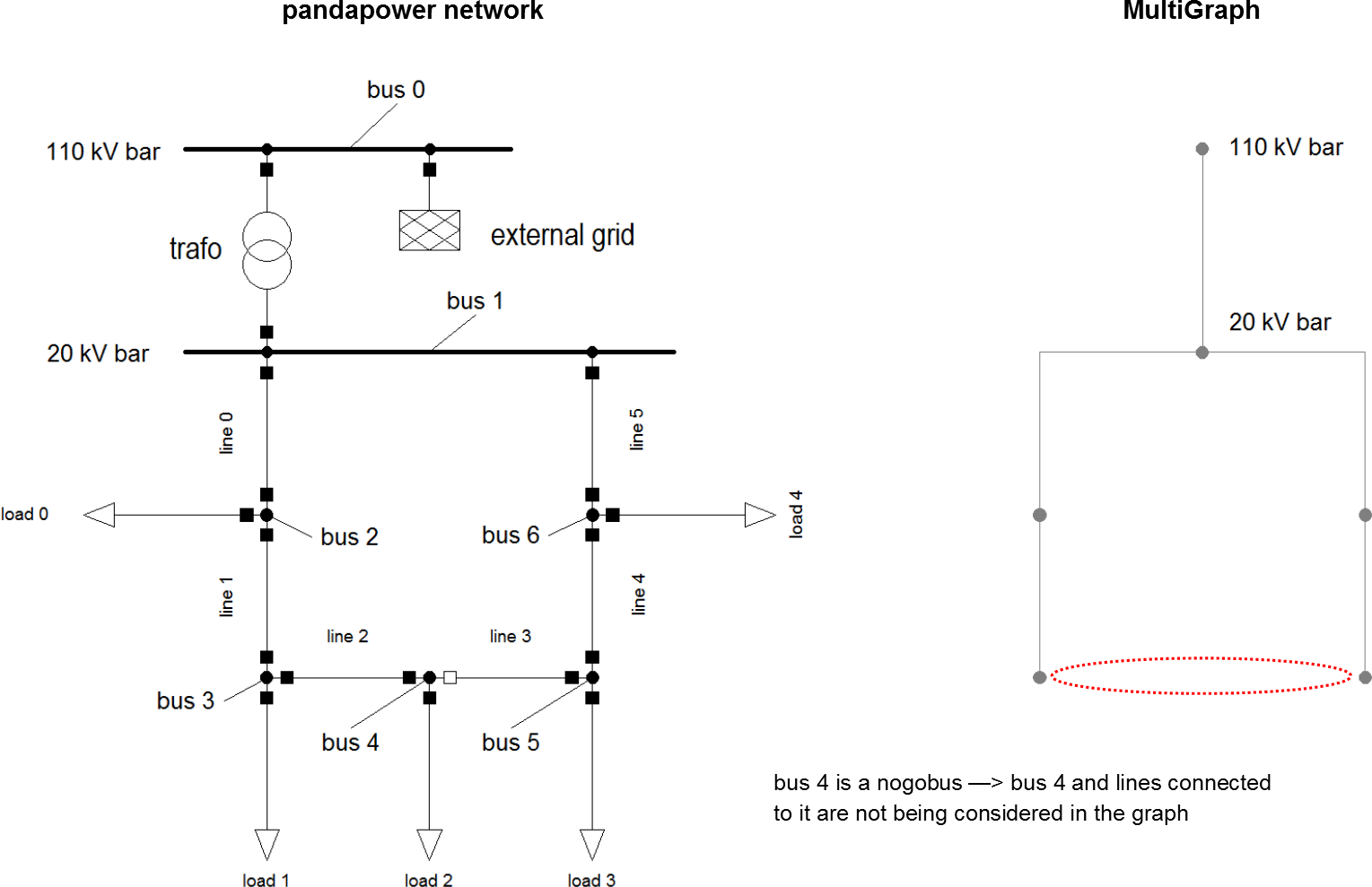create_nxgraph(net, notravbuses = )